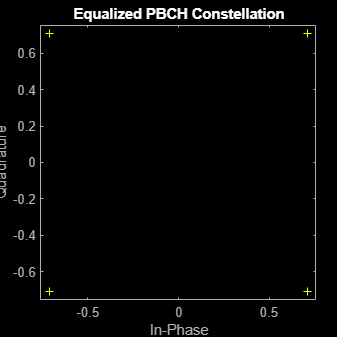Extract PBCH Symbols and Channel Estimates for Decoding

Extract physical broadcast channel (PBCH) symbols from a received grid and associated channel estimates in preparation for decoding a beamformed PBCH.

PBCH Coding and Beamforming

Create a random sequence of binary values corresponding to a BCH codeword. The length of the codeword is 864, as specified in TS 38.212 Section 7.1.5. Using the codeword, create symbols and indices for a PBCH transmission. Specify the physical layer cell identity number.

E = 864;
cw = randi([0 1],E,1);
ncellid = 17;
v = 0;
pbchTxSym = nrPBCH(cw,ncellid,v);
pbchInd = nrPBCHIndices(ncellid);

Use nrExtractResources to create indices for the two transmit antennas of a beamformed PBCH. Use these indices to map the beamformed PBCH into the transmitter resource array.

carrier = nrCarrierConfig('NSizeGrid',20);
P = 2;
txGrid = nrResourceGrid(carrier,P);
F = [1 1i];
[~,bfInd] = nrExtractResources(pbchInd,txGrid);
txGrid(bfInd) = pbchTxSym*F;

OFDM modulate the PBCH symbols mapped into the transmitter resource array.

txWaveform = nrOFDMModulate(carrier,txGrid);

PBCH Transmission and Decoding

Create and apply a channel matrix to the waveform. Receive the transmitted waveforms.

R = 3;
H = dftmtx(max([P R]));
H = H(1:P,1:R);
H = H/norm(H);
rxWaveform = txWaveform*H;

Create channel estimates including beamforming.

hEstGrid = repmat(permute(H.'*F.',[3 4 1 2]),[240 4]);
nEst = 0;

Demodulate the received waveform using orthogonal frequency division multiplexing (OFDM).

rxGrid = nrOFDMDemodulate(carrier,rxWaveform);

In preparation for PBCH decoding, extract symbols from the received grid and the channel estimate grid.

[pbchRxSym,pbchHestSym] = nrExtractResources(pbchInd,rxGrid,hEstGrid);
figure;
plot(pbchRxSym,'o:');Equalize the symbols by performing MMSE equalization on the extracted resources. Plot the results.

pbchEqSym = nrEqualizeMMSE(pbchRxSym,pbchHestSym,nEst);
figure;
plot(pbchEqSym,'o:');
title('Equalized PBCH Constellation');Retrieve soft bits by performing PBCH decoding on the equalized symbols.

pbchBits = nrPBCHDecode(pbchEqSym,ncellid,v)
pbchBits = 864×1
1010 ×

-2.0000
-2.0000
2.0000
-2.0000
-2.0000
2.0000
2.0000
-2.0000
-2.0000
-2.0000
⋮